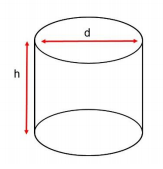## Pre-Lab Assignment

Note: this pre-lab must be finished before you come to lab.

Fill in the blanks on the following table using appropriate SI units:

 Measurement Unit Symbol 1. Length Meter _______ 2. Mass _______ kg 3. Volume _______ L 4. Temperature Kelvin _______ 5. _______ Second s

Fill in the blanks on the following table giving commonly used conversion factors:

Base Unit Prefix Conversion Factor Numerical Form Conversion Factor Scientific Notation
EX. Meter (m) milli (mm) 1 m=1,000 mm 1m=103 mm
6. Liter (L) 1 L=1,000000 μL 1m=106 μL
7. Seconds (s) Centi (c) 1 s=100 cs
8. Grams (g) Kilo (k) 1g=10-3 kg or 1000 g=1 kg
9. Meter (m) Nano (n) 1 m = 1,000,000,000 nm
10. Mol (mol) Milli (m) 1mol=103 mmol

## Experimental Data and Results

*Be sure to answer all questions using the correct number of significant figures. Include all work, units and write answers in scientific notation (if applicable) for full credit.

1. Obtain a thermometer and record the temperature of the room to the correct number of significant figures.

Use a ruler from your drawer to determine the dimensions of a 250 mL beaker. Make sure you use the appropriate number of significant figures. You must show all calculations and units for full credit.

3. Height (measure internal height only). ______________4. Using a ruler and a beaker to determine the volume of solution the beaker can hold. V = πr2h (Remember that cm3 = mL)

5. Why does the beaker capacity exceed the volume printed on the side?

6. Compare the graduated cylinder to the beaker. Which will make the more accurate measurement? Why?

7. Obtain a 50 mL beaker and fill to the 30.0 mL mark using DI water. Make sure you remember to read from the bottom of your meniscus line. Pour this entire amount into your 50 mL graduated cylinder. Read the volume again (using your meniscus). Do not pour out. Record result. ______________________________

8. Using the balance, place a weight boat on the balance and tare or zero. Add the paperclip and record the weight in grams. __________________________. Convert this weight to milligrams ________________________.

9. Again place the weight boat on the balance and tare. Obtain a pipet bulb from your drawer. Using the DI water from your graduated cylinder and a pipet, draw 1mL of DI water by rolling the wheel allowing the water to fill to the mark. Transfer the entire contents of the pipet to the weight boat to determine the mass. Record your results ____________________________. Did 1 mL of water = 1g? Explain your findings.

10. Convert your above result from grams to milligrams.

## Post-Lab Questions

1. How many centimeters are in three meters?

2. A line 20 millimeters long is how many centimeters long?

3. If a student is one meter tall. What is its height in kilometers?

4. How many milliliters are in a two liter bottle?

5. Convert 3.25 centimeters into kilometers

6. Convert 950 g to kg.

7. Convert 275 mm to cm.

8. Convert 1.0 x 103 mL to L.

9. Convert 25 cm to mm.

10. Convert 0.075 m to cm.

11. Convert 15 kg to mg.

12. Convert 3.25 hours to seconds.

13. Convert 2.5 yards to inches. (Remember, there are 3 feet in 1 yard.)

14. Convert 1.2 years into hours. (Remember, there are 365 days in 1 year.)

15. How many centimeters are in 50,555 micrometers?

16. Convert 7.3 x 10-3 km to m.

17. Convert 67.5 millimoles to moles

18. An average human lives to be 70 years old. How many seconds would have elapsed during this person’s lifetime?

19. The usual aspirin tablet contains 5.00 grains of aspirin. How many mg of aspirin are in one tablet? (Remember 1 grain = 1.43 x 10-4 lb, 1 lb = 454 g.)

20. How many 3 oz oranges would you need to eat to meet the U.S. recommended daily allowance of 60.0 mg of vitamin C, if there are 70 mg of vitamin C for every 100 g of orange? (Remember, 16 oz = 454 g.)